# CBSE Previous Year Question Papers Class 12 Chemistry With Solutions

Here we are providing CBSE Previous Year Question Papers Class 6 to 12 solved with soutions CBSE Previous Year Question Papers Class 12 Chemistry with Solutions Chemistry sample paper class 12, Chemistry previous year question paper class 12, cbse class 12 Chemistry sample paper, cbse class 12 Chemistry sample paper 2020, Chemistry sample paper class 12 2020, cbse sample paper 2020 class 12 Chemistry, class Practice of previous year question papers and sample papers protects each and every student to score bad marks in exams.If any student of CBSE Board continuously practices last year question paper student will easily score high marks in tests. Fortunately earlier year question papers can assist the understudies with scoring great in the tests. Unraveling previous year question paper class 12 Chemistry is significant for understudies who will show up for Class 12 Board tests.

## Class 12 Subject Chemistry Paper Set 3 with Solutions

SECTION A

Read the given passage and answer the questions number 1 to 5 that follow :

The d-block of the periodic table contains the elements of the groups 3 – 12 and are known as transition elements. In general, the electronic configuration of these elements is (n – 1) d1–10 ns1–2. The d-orbitals of the penultimate energy level in their atoms receive electrons giving rise to the three rows of the transition metals i.e., 3d, 4d and 5d series. However, Zn, Cd and Hg are not regarded as transition elements. Transition elements exhibit certain characteristic properties like variable oxidation states, complex formation, formation of coloured ions and alloys, catalytic activity, etc. Transition metals are hard (except Zn, Cd and Hg) and have a high melting point.

Question 1: (Marks 1)

Why are Zn, Cd and Hg non-transition elements ?

Answer :

Zn , Cd and Hg have completely filled d10 configuration in their ground state as well as in their oxidized state.

Question 2: (Marks 1)

Which transition metal of 3d series does not show variable oxidation states ?

Answer :

Scandium / Sc

Question 3: (Marks 1)

Why do transition metals and their compounds show catalytic activity ?

Answer :

Because of multiple oxidation states / ability to form complexes / having large surface area

Question 4: (Marks 1)

Why are melting points of transition metals high ?

Answer :

Involvement of (n-1)d and ns electrons in inter atomic metallic bonding / strong metal-metal bonding.

Question 5: (Marks 1)

Why is Cu2+ ion coloured while Zn2+ ion is colourless in aqueous solution ?

Answer :

Presence of unpaired eshowing d-d transition in Cu2+ , while in Zn2+ there is no unpaired electron.

Questions number 6 to 10 are one word answers :

Question 6: (Marks 1)

Name the cell which was used in the Apollo Space Programme.

Answer :

H2 - O2 Fuel cell

Question 7: (Marks 1)

How many coulombs are required for the oxidation of 1 mol of H2O to O2?

Answer :

2 x 96500 C / 193000 C

Question 8: (Marks 1)

Write the slope value obtained in the plot of ln[R] vs. time for a first order reaction.

Answer :

Slope = -k

Question 9: (Marks 1)

Name the disaccharide which on hydrolysis gives two molecules of glucose.

Answer :

Maltose

Question 10: (Marks 1)

Name the class of the synthetic detergent which is used in toothpaste.

Answer :

Anionic detergent

Questions number 11 to 15 are multiple choice questions :

Question 11: (Marks 1)

Which of the following is refined by the zone refining process ?

(A) Cu

(B) Zn

(C) Ge

(D) Sn

Answer :

(C) Ge

Question 12: (Marks 1)

Racemisation occurs in

(A) SN2 reaction

(B) SN1 reaction

(C) Neither SN2 nor SN1 reactions

(D) SN2 reaction as well as SN1 reaction

Answer :

(C) Neither SN2 nor SN1 reactions

Question 13: (Marks 1)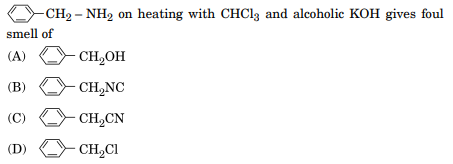Answer :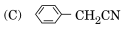Question 14: (Marks 1)

One mole of CrCl3. 6H2O compound reacts with excess AgNO3 solution to yield two moles of AgCl (s). The structural formula of the compound is

(A) [Cr(H2O)5 Cl] Cl2. H2O

(B) [Cr(H2O)3 Cl3] . 3H2O

(C) [Cr(H2O)4 Cl2] Cl . 2H2O

(D) [Cr(H2O)6] Cl3

Answer :

(A) [Cr(H2O)5 Cl] Cl2. H2O

Question 15: (Marks 1)

Peptide linkage is present in

(A) Carbohydrates

(B) Vitamins

(C) Proteins

(D) Rubber

Answer :

(C) Proteins

For questions number 16 to 20, two statements are given - one labelled Assertion (A) and the other labelled Reason (R). Select the correct answer to these questions from the codes (i), (ii), (iii) and (iv) as given below :

(i) Both Assertion (A) and Reason (R) are correct statements, and Reason (R) is the correct explanation of the Assertion (A).

(ii) Both Assertion (A) and Reason (R) are correct statements, but Reason (R) is not the correct explanation of the Assertion (A).

(iii) Assertion (A) is correct, but Reason (R) is incorrect statement.

(iv) Assertion (A) is incorrect, but Reason (R) is correct statement.

Question 16: (Marks 1)

Assertion (A) : Conductivity of an electrolyte decreases with decrease in concentration.

Reason (R) : Number of ions per unit volume increases on dilution.

Answer :

(iii) Assertion (A) is correct, but Reason (R) is incorrect statement.

Question 17: (Marks 1)

Assertion (A) : The C – O – H bond angle in alcohols is slightly less than the tetrahedral angle.

Reason (R) : This is due to the repulsive interaction between the two lone electron pairs on oxygen.

Answer :

(i) Both Assertion (A) and Reason (R) are correct statements, and Reason (R) is the correct explanation of the Assertion (A).

Question 18: (Marks 1)

Assertion (A) : [Pt(en)2 Cl2]2+ complex is less stable than [Pt(NH3)4Cl2]2+ complex.

Reason (R) : [Pt(en)2Cl2]2+ complex shows chelate effect.

Answer :

(iv) Assertion (A) is incorrect, but Reason (R) is correct statement.

Question 19: (Marks 1)

Assertion (A) : Osmotic pressure is a colligative property.

Reason (R) : Osmotic pressure is directly proportional to molarity.

Answer :

(i) Both Assertion (A) and Reason (R) are correct statements, and Reason (R) is the correct explanation of the Assertion (A).

Question 20: (Marks 1)

Assertion (A) : Reactivity of ketones is more than aldehydes.

Reason (R) : The carbonyl carbon of ketones is less electrophilic as compared to aldehydes.

Answer :

(iv) Assertion (A) is incorrect, but Reason (R) is correct statement.

SECTION B

Question 21: (Marks 2)

In the given reaction

A + 3B → 2C,

the rate of formation of C is 2·5 x 10–4 mol L–1 s–1.

Calculate the

(i) rate of reaction, and

(ii) rate of disappearance of B.

Answer :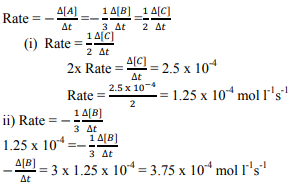Question 22: (Marks 2)

Write the role of the following :

(i) NaAlF4 in the extraction of Aluminium

(ii) CO in the refining of Ni

OR

Write the chemical equations involved in the leaching of bauxite ore to prepare pure alumina.

Answer :

i) Acts as solvent / lowers the melting point of the mixture / Increases the conductivity.

ii) It forms a volatile compound Ni(CO)4, which decomposes at higher temperature to give pure Nickel.

OR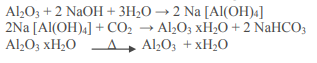Question 23: (Marks 2)

Write two differences between physisorption and chemisorption.

OR

Define the following terms with a suitable example of each :

(i) Associated colloids

(ii) O/W emulsion

Answer :Question 24: (Marks 2)

(a) Write the IUPAC name and hybridisation of the complex [CoF6]3–. (Given : Atomic number of Co = 27)

(b) What type of isomerism is shown by the complex [Co(en)2Cl2]2+? Name the structure of an isomer of this complex which is optically active.

Answer :

a) Hexafluoridocobaltate(III)

sp3d2

b) Isomerism – Geometrical / optical cis isomer is optically active

Question 25: (Marks 2)

Give reasons :

(i) Shaving soaps contain glycerol.

(ii) Antacids should not be used for longer time.

Answer :

(i) To prevent rapid drying.

(ii) Antacids will make the stomach alkaline and trigger the production of more acid.

Question 26: (Marks 2)

Define the following terms :

(i) Oligosaccharides

(ii) Invert sugar

Answer :

i) Carbohydrates that yield two to ten monosaccharides units on hydrolysis.

ii) Hydrolysis of sucrose brings about a change in the sign of rotation, from dextro (+) to laevo (-) and the product is named as invert sugar.

Question 27: (Marks 2)

Write the products of the following reaction :

6 XeF4 + 12 H2O →

Is this reaction a disproportionation reaction ? Give reasons in support of your answer.

Answer :

6XeF4 + 12 H2O → 4Xe + 2XeO3 + 24HF + 3O2

Yes

Xe+4 changes to Xe o and Xe +6 / Xe gets oxidized as well as reduced in the same reaction.

SECTION C

Question 28: (Marks 3)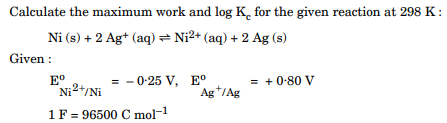Answer :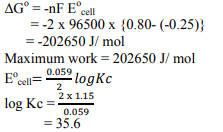Question 29: (Marks 3)

A first order reaction is 40% complete in 80 minutes. Calculate the value of rate constant (k). In what time will the reaction be 90% completed ?

[Given : log 2 = 0·3010, log 3 = 0·4771, log 4 = 0·6021, log 5 = 0·6771, log 6 = 0·7782]

Answer :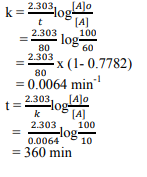Question 30: (Marks 3)

Write the names and structures of the monomers in the following polymers :

(i) Buna-S

(ii) Nylon-6,6

(iii) Bakelite

Answer :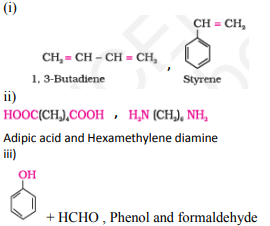Question 31: (Marks 3)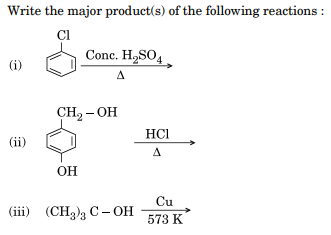Answer :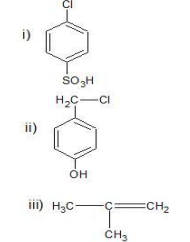Question 32: (Marks 3)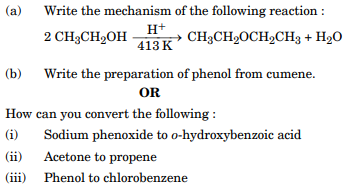Answer :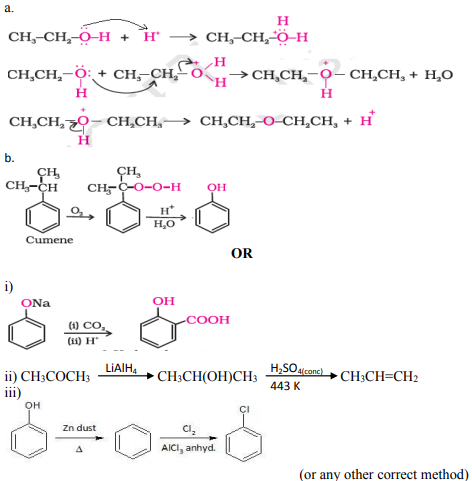Question 33: (Marks 3)

Write the products formed when (CH3)3 C – CHO reacts with the following reagents :

(i) CH3COCH3 in the presence of dilute NaOH

(ii) HCN

(iii) Conc. NaOH

Answer :

i) (CH3)3CCH(OH)CH2COCH3

ii) (CH3)3CCH(OH)CN

iii) (CH3)3CCOONa + (CH3)3CCH2OH

Question 34: (Marks 3)

Define Lyophobic and Lyophilic sol with a suitable example of each. Why is coagulation of Lyophilic sol difficult as compared to Lyophobic sol ?

OR

Define the following terms :

(i) Shape-selective catalysis

(ii) Kraft temperature

(iii) Peptization

Answer :

Lyophobic sol is liquid or water repelling.

Example: Fe(OH)3 sol / gold sol (or any other suitable example)

Lyophilic sol is liquid or water attracting. Example: starch sol / rubber sol (or any other suitable example)

Due to the charge and solvation of the colloidal particles.

OR

i) The catalytic reaction that depends upon the pore structure of the catalyst and the size of the reactant and product molecules.

ii) The formation of micelles takes place only above a particular temperature is called Kraft temperature (Tk)

iii) Process of converting a precipitate into colloidal sol by shaking it with dispersion medium in the presence of a small amount of electrolyte.

SECTION D

Question 35: (Marks 5)

(a) Give reasons :

(i) Helium does not form compounds like Xenon.

(ii) HClO4 is a stronger acid than HOCl.

(iii) Sulphur is a polyatomic solid whereas Oxygen is a diatomic gas.

(b) Write one reaction as an example of each, to show that conc. H2SO4 acts as

(i) an oxidising agent, and

(ii) a dehydrating agent.

OR

(a) Account for the following :

(i) Hydration enthalpy of F ion is more than Cl ion.

(ii) SO2 is a reducing agent, whereas TeO2 is an oxidising agent in group-16 oxides.

(b) Write the reaction of F2 with water. Why does I2 not react with water ?

(c) Draw the structure of XeF2 .

Answer :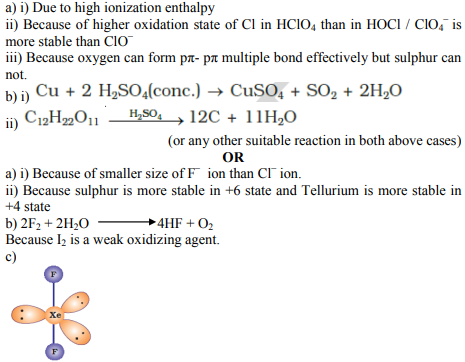Question 36: (Marks 5)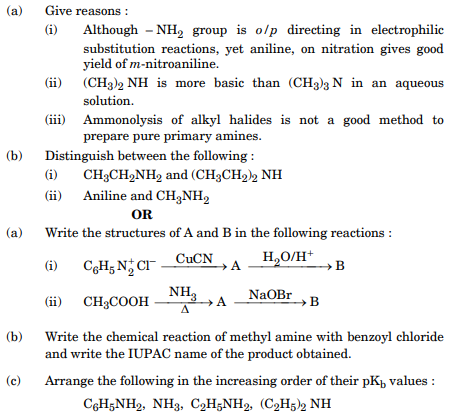Answer :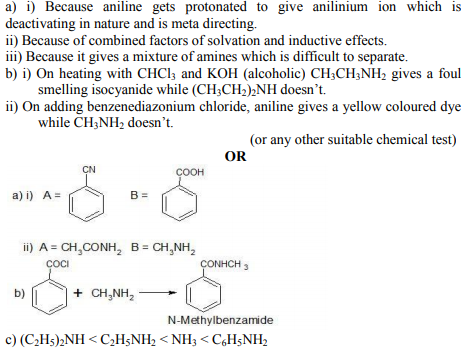Question 37: (Marks 5)

(a) A solution contains 5·85 g NaCl (Molar mass = 58·5 g mol–1) per litre of solution. It has an osmotic pressure of 4·75 atm at 27°C. Calculate the degree of dissociation of NaCl in this solution.

(Given : R = 0·082 L atm K–1 mol–1)

(b) State Henry’s law. Why is air diluted with helium in the tanks used by scuba divers ?

OR

(a) When 19·5 g of F – CH2 – COOH (Molar mass = 78 g mol–1) is dissolved in 500 g of water, the depression in freezing point is observed to be 1°C. Calculate the degree of dissociation of F – CH2 – COOH.

[Given : Kf for water = 1·86 K kg mol–1]

(b) Give reasons :

(i) 0·1 M KCl has higher boiling point than 0·1 M Glucose.

(ii) Meat is preserved for a longer time by salting.

Answer :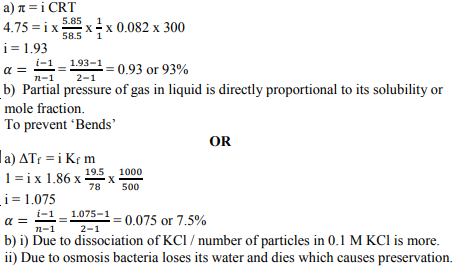☞ Click here for privious year Question papers

## Chemistry sample paper class 12, Chemistry previous year question paper class 12, cbse class 12 Chemistry sample paper, cbse class 12 Chemistry sample paper 2020, Chemistry sample paper class 12 2020, cbse sample paper 2020 class 12 Chemistry, class 12 Chemistry sample paper 2020, class 12 important questions Chemistry, cbse class 12 board exam Chemistry paper, Chemistry previous year question papers class 12 with solutions, Chemistry sample paper class 12 2019, cbse class 12 Chemistry question paper 2017 solved pdf, cbse class 12 Chemistry question paper 2018, class 12 Chemistry paper 2019, Chemistry question paper for class 12, cbse class 12 Chemistry paper 2019

### NCERT Books Free Pdf Download for Class 5, 6, 7, 8, 9, 10 , 11, 12 Hindi and English Medium

 Mathematics Biology Psychology Chemistry English Economics Sociology Hindi Business Studies Geography Science Political Science Statistics Physics Accountancy

## Please Share this webpage on facebook, whatsapp, linkdin and twitter.

Copyright @ ncerthelp.com A free educational website for CBSE, ICSE and UP board.